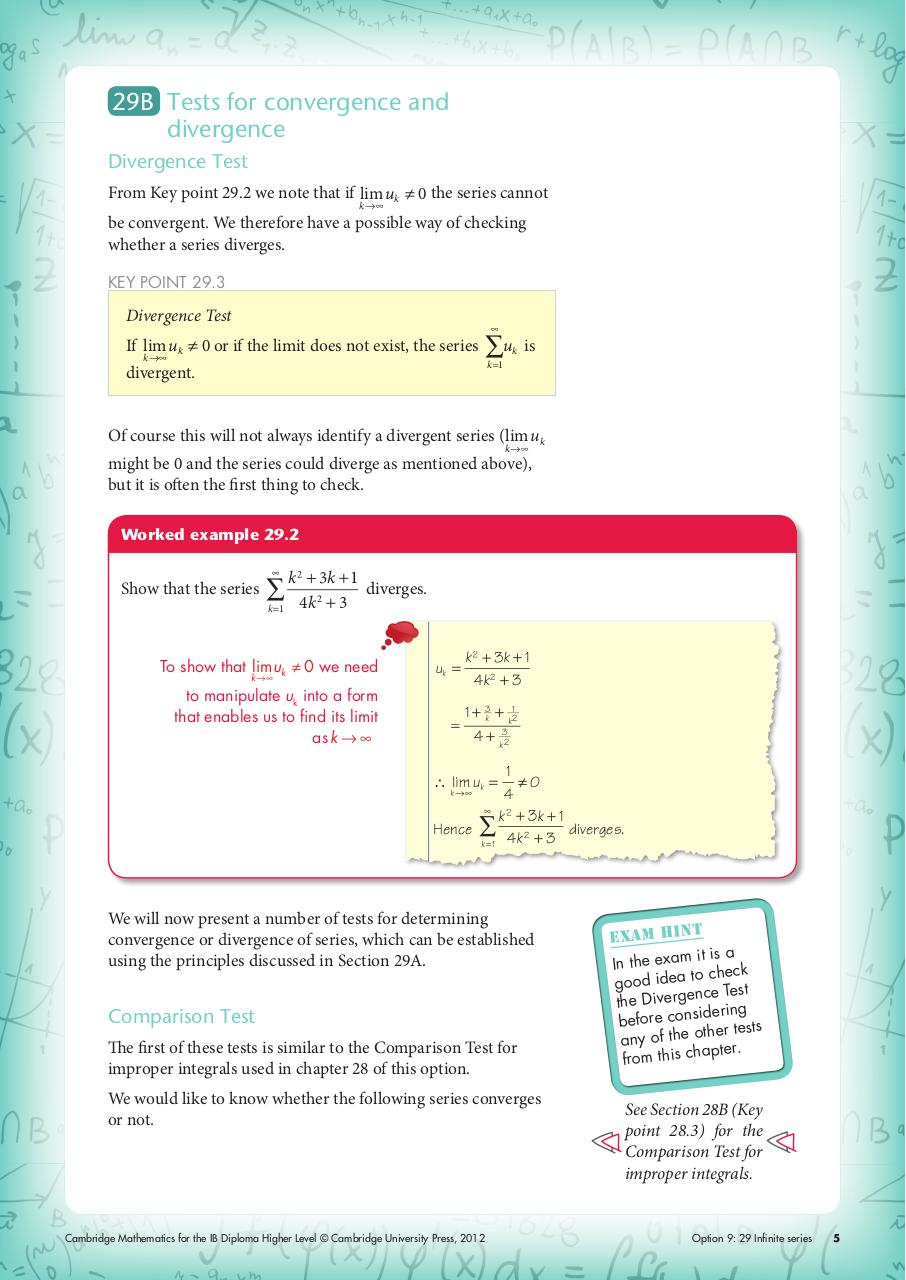# ch12.pdfPage 1 23426

#### Text preview

29B Tests for convergence and
divergence
Divergence Test
From Key point 29.2 we note that if lim uk ≠ 0 the series cannot
k →∞

be convergent. We therefore have a possible way of checking
whether a series diverges.
KEY POINT 29.3

Divergence Test
If lim uk ≠ 0 or if the limit does not exist, the series
k →∞

∑u

k

is

k =1

divergent.

Of course this will not always identify a divergent series (lim uk
k →∞

might be 0 and the series could diverge as mentioned above),
but it is often the first thing to check.
Worked example 29.2

Show that the series

k =1

k

3k + 1
diverges.
4k 2 + 3

To show that lim uk ≠ 0 we need

uk =

to manipulate uk into a form
that enables us to find its limit
as k → ∞

=

k →∞

k 2 3k + 1
4k 2 + 3
1 + 3k + k12
4 + k32

1
≠0
4

k 2 3k + 1
Hence ∑
diverges.
2
k = 1 4k + 3
∴ lim uk =
k →∞

We will now present a number of tests for determining
convergence or divergence of series, which can be established
using the principles discussed in Section 29A.

Comparison Test
The first of these tests is similar to the Comparison Test for
improper integrals used in chapter 28 of this option.
We would like to know whether the following series converges
or not.

Cambridge Mathematics for the IB Diploma Higher Level © Cambridge University Press, 2012

t
exam hin
it is a
In the exam
to check
good idea
nce Test
the Diverge
dering
before consi
ther tests
any of the o
apter.
from this ch

See Section 28B (Key
point 28.3) for the
Comparison Test for
improper integrals.

Option 9: 29 Infinite series

5